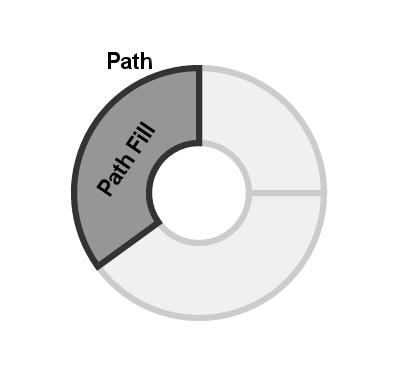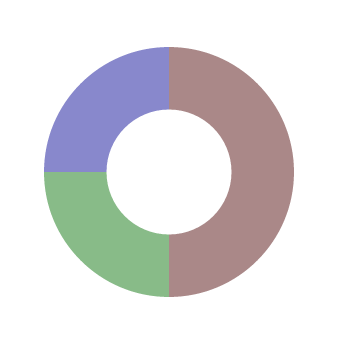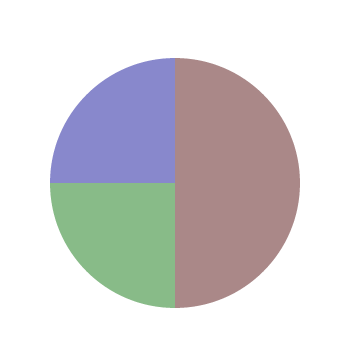## Pie and Donut Charts in D3.js

- October 1, 2013

D3.js is a JavaScript library that is widely used in data visualisation and animation. The power of d3.js and its flexibility, comes at the expense of its steep learning curve. There are some libraries built on top of it that provide numerous off-the-shelf charts in order to make the users’ life easier, however, learning to work with d3.js is essential sometimes, especially when you need to create sophisticated and custom visualisations.

If you are not familiar with d3.js, you can read this introduction. In brief, just like jQuery and other DOM manipulation frameworks, it allows you to dynamically manipulate the properties and attributes of your HTML document elements. Nevertheless, it doesn’t stop here. Its power comes from two additional functionality. It can also create and manipulate SVG elements, and it can also bind the DOM or SVG elements to arrays of data, so that any changes in that data will be reflected on those elements they are binded to. There are numerous SVG shapes, such as circles, rectangles, paths and texts. Those shapes serve as the building blocks of your visualisations. For example, a bar chart is composed of multiple rectangles. while a scatter plot is made of circles scattered in different parts of you drawing area. You may also want to see this interactive tutorial about creating and manipulation SVG elements, as well as binding them to data arrays.In this tutorial, we are going to show how to create pie charts and donut charts, which are very similar to pie charts with only one difference, their centre is hollow. Those two charts are built using SVG paths. The SVG path is a more advanced shape compared to circles and rectangles, since it uses path commands to create any arbitrary shape we want. So, as you can see in the above figure, a donut chart is composed of multiple arc-like paths, with a different fill colour. Fortunately, d3.js provides a helper functions to draw arcs. Arcs are drawn using 4 main parameters: startAngle, endAngle, innerRadius and outerRadius. The angles are given in radians rather than degrees, so a full circle is 2 π instead of 360 degrees. Bear with me for now, and I will show you a way to enter angles using more meaningful ways later on.

To draw an arc, let’s first add the following SVG tag in our html documents:

`<svg id="svg_donut" width="600" height="400"></svg>` Now, to draw an arc that goes from 0 to ¾ of a full rotation, with inner radius of 50 pixels and outer radius of 100 pixels, we need to type the following JavaScript code. `var vis = d3.select("#svg_donut");`
```var arc = d3.svg.arc() .innerRadius(50) .outerRadius(100) .startAngle(0) .endAngle(1.5*Math.PI);``` ```vis.append("path") .attr("d", arc) .attr("transform", "translate(300,200)");``` In the above code, we first select our SVG element using its identifier, “#svg_donut”. We save our selection into a variable called “vis”. d3.svg.arc() is the d3.js helper function that we use rather than the SVG path commands. We give it our previously mentioned 4 parameters and save the result into a variable called “arc”. Now, to actually create the path and append it to our SVG element, we use vis.append(“path”), then assign the “arc” to its “d” attribute. You may consider the “d” attribute as an alternative to the SVG path command. By default, things are drawn in the top left corner of the SVG element. To move the arc to the center of our SVG, we translate it to (300,200), which our width/2 and height/2 respectively.

The output will look as follows:Dealing with radian angles is boring. Fortunately, d3.js provides us with another helper functions called scales. It basically helps us mapping one scale, to another. Let’s say, rather than having the full rotation spanning from 0 to 2 π, we want it to be from 0 to 100. All we need to do is to write the following code:

`var myScale = d3.scale.linear().domain([0, 100]).range([0, 2 * Math.PI]);` From now on, myScale(0) = 0, myScale(100) = 2 π, myScale(75) = 1.5 π, etc. Thus, the above code can be written as follows now. `var vis = d3.select("#svg_donut");`
```var myScale = d3.scale.linear().domain([0, 100]).range([0, 2 * Math.PI]); var arc = d3.svg.arc() .innerRadius(50) .outerRadius(100) .startAngle(myScale(0)) .endAngle(myScale(75));``` ```vis.append("path") .attr("d", arc) .attr("transform", "translate(300,200)");``` So far, we learnt how to create an arc. Pie or donut charts are composed of multiple arcs the angle of each of them represents the value of one item of our data to the overall data values. In other words if we have 3 mobile brands, A, B and C, where A has 50% of the market share, while B and C, have 25% each. Then we represent them in our charts as 3 arcs with angles of π, π/2 and π/2 respectively. To implement this using the d3.js way, you need to have an array of data and bind it to arcs. As you have seen in the aforementioned tutorial, SVG elements can be created on the fly to match the data they are binded to. `var cScale = d3.scale.linear().domain([0, 100]).range([0, 2 * Math.PI]);` `data = [[0,50,"#AA8888"], [50,75,"#88BB88"], [75,100,"#8888CC"]]` `var vis = d3.select("#svg_donut");` ```var arc = d3.svg.arc() .innerRadius(50) .outerRadius(100) .startAngle(function(d){return cScale(d);}) .endAngle(function(d){return cScale(d);});``` ```vis.selectAll("path") .data(data) .enter() .append("path") .attr("d", arc) .style("fill", function(d){return d;}) .attr("transform", "translate(300,200)");``` The data array is composed of 3 data items, each of them is given as an array of 3 values. The first item goes from 0 to 50% of the donut with a background colour of “#AA8888”, the starting angle of the second item should comes right after the first, i.e. 50%, and it goes to 75%, with a background colour of “#88BB88. Similarly, the third item goes from 75% to 100%, with a background colour of “#8888CC”. To append arcs dynamically based on our data, we select path, bind our data to the selections then append new paths accordingly: `vis.selectAll("path").data(data).enter().` The “startAngle”, “endAngle” and “fill” are grabbed from the data items as shown in red. The resulting donut looks as follows:The exact same code can be used to create a pie chart, the only difference is that the value given to the innerRadius() should be set to 0. The resulting pie chart can also be seen below:Between you and me, there are existing libraries built on top of d3.js than can create pie charts for you with less code. So, why do you need to learn all this? The idea here is to learn the d3.js internal, so you can tweak the above code to suite your needs. An off-the-shelf library can give you a pie chart, or a donut chart. But what about the following charts?